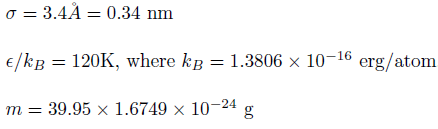# [lammps-users] UNITS lj

Hello,

i have a question: i want to convert time ftom lj units to time in seconds.

i have: tau = t* = t (epsilon / m / sigma^2)^1/2 ,as i understood, using this formula i will get time in femtoseconds?
What is a value “t” means in this formula? What does it equal?
Explain, please, i’m confused a little.You have to use consistent units. Combining nm, erg/atom, and g will get you something, but it’s almost certainly not going to be femtoseconds!

Your best bet is to write everything in terms of standard metric units. That way, you know the result should be in seconds. Note also that the term gives you the value of t in terms of dimensionless units. Also keep in mind that the expression tells you how to multiply the time t to get a dimensionless time tau. Thus, the basic unit of time is therefore sqrt((m * sigma^2) / epsilon), and your timestep is whatever fraction of that you specify in the “timestep” command.

–AEIHello,

i have a question: i want to convert time ftom lj units to time in seconds.

i have: tau = t* = t (epsilon / m / sigma^2)^1/2 ,as i understood, using this formula i will get time in femtoseconds?
What is a value "t" means in this formula? What does it equal?
Explain, please, i'm confused a little.

if you are so desperate to get "real" units, why
don't you just use them in your input right away?
that'll avoid the mess you created below entirely.

cheers,
axel.

t is the value of time in actual units; t* has no units.

Steve